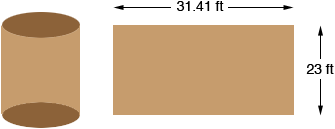SEARCH HOMEMath Central Quandaries & QueriesQuestion from jack: i'm looking for the square footage of a tank that is 10'diameter x 23'high,floor,ceiling and wallsHi Jack,

You can think of the tank as being constructed from three pieces, two circular disks that form the floor and ceiling and a rectangle that is rolled to form the wall. Since the diameter is 10 feet the radius r is 10/2 = 5 feet. The area of a circular disk is π r2 so each disk has an area of π × 52 = 78.54 square feet.The length of the rectangle is the circumference of the circle which is 2 π r = 31.41 feet. Thus the area of the rectangle is 23 × 31.41 = 722.57 square feet. Thus the total surface area is

2 × 78.54 + 722.57 = 879.6 square feet.

PennyMath Central is supported by the University of Regina and The Pacific Institute for the Mathematical Sciences.